GNSS Geodesy Innovation Interviews UAV/UAS Positioning Surveying Applications Perspective Time Navigation Mapping Policy Land Administration News Archives LBS GIS SDI Imaging E-Zine
 Mycoordinates

# High-piled wharf–deformation prediction

Sep 2009 | Comments Off on High-piled wharf–deformation prediction

###### On the basis of the Back Propagation neural network and its training method the known data was used in the paper to establish a computation model with Back Propagation neural network to monitor the deformation of the 14th berth of No.2 harbor-pool of Tianjin port

The high pile wharf has the advantage of slight wave reflection, steady anchoring condition, little investment and the construction time being short. Therefore it has been widely used in coastal areas where the ground has soft soil. But, on the other hand its penetrant structure raises some problems also for the structure. In order to guarantee the request of ships draft, the front water area of the wharf has to be dug more deep, and the rear area of the wharf needs massive backfill so as to link it with land, which can lead to the balance of soil body being destroyed, and may cause deformation of the bank slope which would influence the stability of the high wharf pile foundation. Thus, if no control measures are carried out (such as deformation monitor forecast), there would be an enormous security hidden danger in the wharf.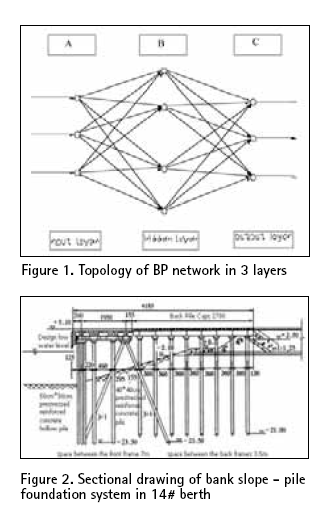Regarding the wharf deformation monitor forecast, the traditional analysis models include the random model, deterministic model and the mix model. But in case of complex influencing factors, it is beyond the ability of the traditional analysis models to describe the non-linear mapping relations between the wharf structure and their deformation, further it affects the data fitting and the precision of deformation forecast.

The artificial neural network model may solve this problem to a great extent with its splendid self-organization, auto-adaptability, fuzzy judgment and inference, and better self-study ability. At present, the BP neural network model has been widely applied in dam examination forecast. This paper establishes an improved BP neural network model used for the deformation observation data analysis with the high pile wharf deformation forecasts system. The BP network is the forward feed network which is composed by a nonlinear transformation unit, and is one kind of teacher’s studying algorithm. It revises the connection weight between layers and the threshold value according to the actual output error, making the value of exports and actual value as close as possible, thus enabling the forecast to have good accuracy.

## The Principle and algorithm of ANN

BP (Back Propagation) network The BP neural network usually contains three parts the input layer, the hidden layer and the output layer. The hidden layer may contain one or more layers, and each layer is composed of many neurons. Its network topology is shown in Figure1. Its characteristic is that: the neuron in each layer only connects with the neuron in the neighboring layer; there is no neuron connection within the layer; and there is no non-feedback connection between each neuron layer as well. Firstly, the input signal is transmitted forward to the hidden node, and then with the transition function, the information of hidden node is transmitted to output node where the result should be output after the processing. The transmitting function used in node usually selects the Sigmoid function. As a general rule, the hidden layer uses the S logarithm or the tangent activation function, but output layer selects the linear activation function.

## The algorithm of BP neural network study

The BP neural network uses the algorithm of error reverse-transmit study with the technology of gradient searching. It realizes the minimum of mean-square deviation between the actual network output and the expectant output. The procession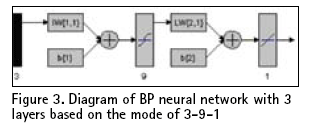of the network study is the one of error transmitting backward on one hand while revising the weight on the other hand. The study process in the network is composed by forward-transmitting and reverse transmitting. In the forward process, the input signal dealt in each layer begins from the input layer to output layer, and the neuron condition in each layer only influences the next layer. If the expectant output cannot be obtained in output layer, the transmitting changes into reverse which will make the output error return along the original connection circuit. Through revising each neuron layer of the weight, the signal error will become minimized. After obtaining the appropriate network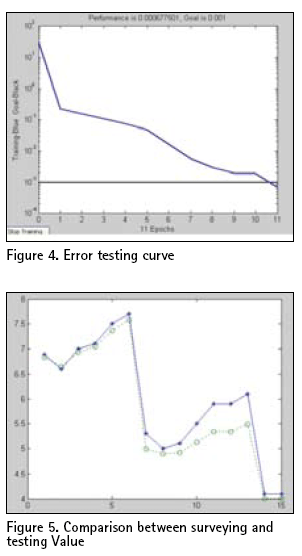of new sample may be carried on.

## Information forward transmission

Supposing BP network has L layers, for P the assigned sample and the network expecting output is Td=[Td1,Td2,……,Tdp]

When the Pth sample is inputted, the operating characteristic of jth neuron in l (l=1,2, ……L-1) layer is: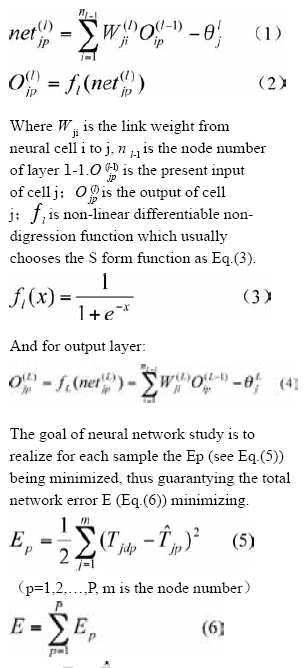2.2.2. Strive for the weight change and the erroneous reverse dissemination using the gradient drop law

When revising the value of network weight and threshold using the gradient drop law, the iteration equation of weight coefficient in lth layer is Eq. (7).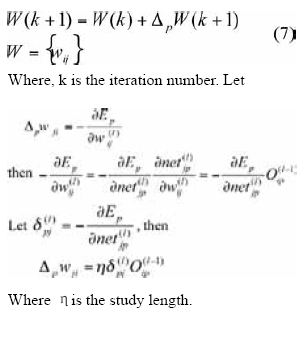The procession of network training

(1) Initializing the value of all connecting weight: The initial weight value to the network with group of random numbers is given. Setting the study length being ?, the allowance error e and the network structure (i.e. network layer number L and each node number nl). The initial value should generally be set with small random number so as to guarantee that an unusual saturated case does not exist in the network.

(2) When the appropriate training sample is selected, the sample data are inputted to the network so as to acquire the output value of the network.

(3) After the deviation d(l) jp between expected value and output value of sample is computed, each layer weight towards the direction of reducing deviation has been adjusted with the method of gradient drop algorithm from the output layer to the input layer.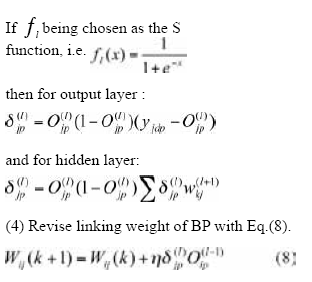Where k is the studying number, ? is the studying factor. More the value of ?, the fiercer is the weight change, which could lead the studying process to vibrate. Therefore, in order to get the value of studying factor large enough but so as to not lead to vibration, an additional actional value should be added in the weight correlation formula. (5)Each group data of the training sample should be carried on the training until the entire training deviation meets the accepted degree. The neural network being trained could express accurately the relationship between the input and the output. When the known group is inputted, the output value could be acquired using this neural network.

## BP network applying in deformation forecast of high pile wharf

This paper takes 14# berth bank slope of 2# harbor of the high pile wharf basin in the Tianjin Port as an example to carry on the simulation forecast analysis. The horizontal deformation of the high pile wharf based on the soft soil ground is caused by 1 or 2 main factors and many additional factors, the main factors are: the strength of the high pile wharf rear-area carries and the ships lashing rope strength as well as the earth property of pierre-perdue dike. Therefore, we can take the earth elasticity coefficient and the strength of the high pile wharf reararea carries with the horizontal lashing rope strength of ships as the neural network input parameter; the wharf horizontal deformation as the network output, and establish the neural network model, carries on the deformation forecast for the high pile wharf. Based on the analysis mentioned above, three layers BP neural network has been established with the input layer being 3 nodes ( earth elasticity coefficient, high pile wharf rear-area carries, horizontal lashing rope strength), the output layer being 1 node (horizontal deformation) and the hidden layer nodes have yet to be decided. In many cases, the nodes number in hidden layer could be set according to Eq.(9).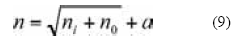Where n is the node number of hidden layer, ni is the node number of input layer, no is the node number of output layer, is a constant between 1 and 10. In the paper, the n scope varies from 3 to 12, the neural network procedure has been written using Matlab. The finally training number is 9000 times, the goal error is 0.001, the node number of hidden layer is 9 with which the goal error and the testing time would achieve optimization. 50 sample points have been selected as the training sample of the network study while another 15 sample points picked as the forecast sample for evaluating the network training effect which are shown in Table 1 and Figure 4 and Figure 5. Seen from Table 1, based on the neural network model, the deviation of horizontal deformation of the high pile wharf in 14# berth between predicted value and actual value is very small, except testing points 11, 12, and 13 (shown in Figure 5).

This is caused primarily by the sample quantity not being enough and therefore the network can not study the relationship between every element, like in real life. Although the generalization ability can not be shown well, but the total relative error may be accepted (Figure 4).

Based on the training network, the input value (earth elasticity coefficient, high pile wharf rear-area carries, horizontal lashing rope strength) is given with the weight and the threshold value which have been saved in the network, thus the deformation value of the high pile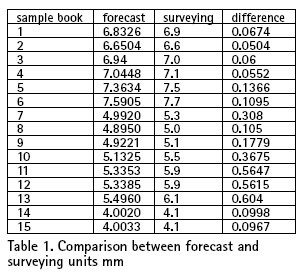wharf structural could be output, and the goal of the high pile wharf structural deformation forecast could be realized.

## Conclusion

(1) The synthetic evaluation for water conservancy project structure quality is the multi index, multi-layers hierarchical analysis. Based on the BP neural network method , a new evaluation method used in the high pile wharf quality synthetic evaluation has been put forward, it is a synthetic evaluation model combining with quality and quantity analysis and even more approaches to human thought pattern. Through the study of expert evaluation mode with assigned sample, expert’s experience, knowledge, subjective judgment the tendency to the important goal have been acquired. When such object needs a synthetic evaluation, this model could reappear the appraising of expert experience knowledge and the intuition thought. So the combination of qualitative and quantitative analysis should be reached which could guarantee objectivity of the appraisal result of such object.

(2) This paper research is aimed at the high pile wharf structure, regarding other object of water transportation engineering structure (such as dam);. just changing the input parameter (or deformation affect factor), the neural network model for object deformation forecast could be established with the same network structure used above.

(3) It is feasible to use fuzzy artificial neural networks for wharf deformation forecast and the forecast error meets the need of hydraulic engineering while the fuzzy uncertainty between deformation factors have been solved. Nonetheless there are many problems that need to be discussed when the artificial neural network is used for the deformation forecast, such as how to use the sample information fully, how to solve various factors weight evaluation and increase the forecast precision etc. Meanwhile the relevance of each influence factor should be analyzed fully so as to reduce the model input parameter and enhance the output stability.

## References

? Jiang shaofei, Structure optimization and damage examination based on neural network, [M], Science Press, 2002

? Chen changlin, Horizontal deformation control for high pile wharf, Water Transport Engineering, [J], 2000, 313(2, 19~20

? Wang xinzhou, Shi wenzhong, Wang shuliang, Fuzzy space information processing, [M], Wuhan University Press, 2003

? Wang xinzhou, Deng xinshen, Dam deformation forecast based on fuzzy neural network model, [J] , Wuhan University journal (information science version), 2003.(2)

? Cehn jiuyu, Lin jian, The mathematics processing of observation data [M], Shanghai Jiao Tong University Press, 1986

? Wuzhongru, Shen changsong, Ruan huanxiang, Hydraulic engineering structure safe monitoring theory and application, [M], Hohai University, Nanjing, 1990

? Chen ping, Yuan menquan, Deformation reason analysis and preventive measure for high pile wharf, China Habour Construction, [J], 146

? Wang yongji, Tu jian, Control of neural cell network, [M], Mechanical Industry Press, 1998

? Yuan zengren, Artificial neural networks and its application, [M], Tsinghua University Press , 1999

? Neural network model and MATLAB simulated program design, [M], Tsinghua University Press , 2006## Xiaodong YI

###### yixiaodongdlut@126.com## Erhu WEI

###### ehwei@sgg.whu.edu.cn## Chengke JIANG

##### INDUSTRY | LBS | GPS | GIS | REMOTE SENSING | GALILEO UPDATE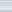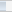(3 votes, average: 4.33 out of 5)Loading...